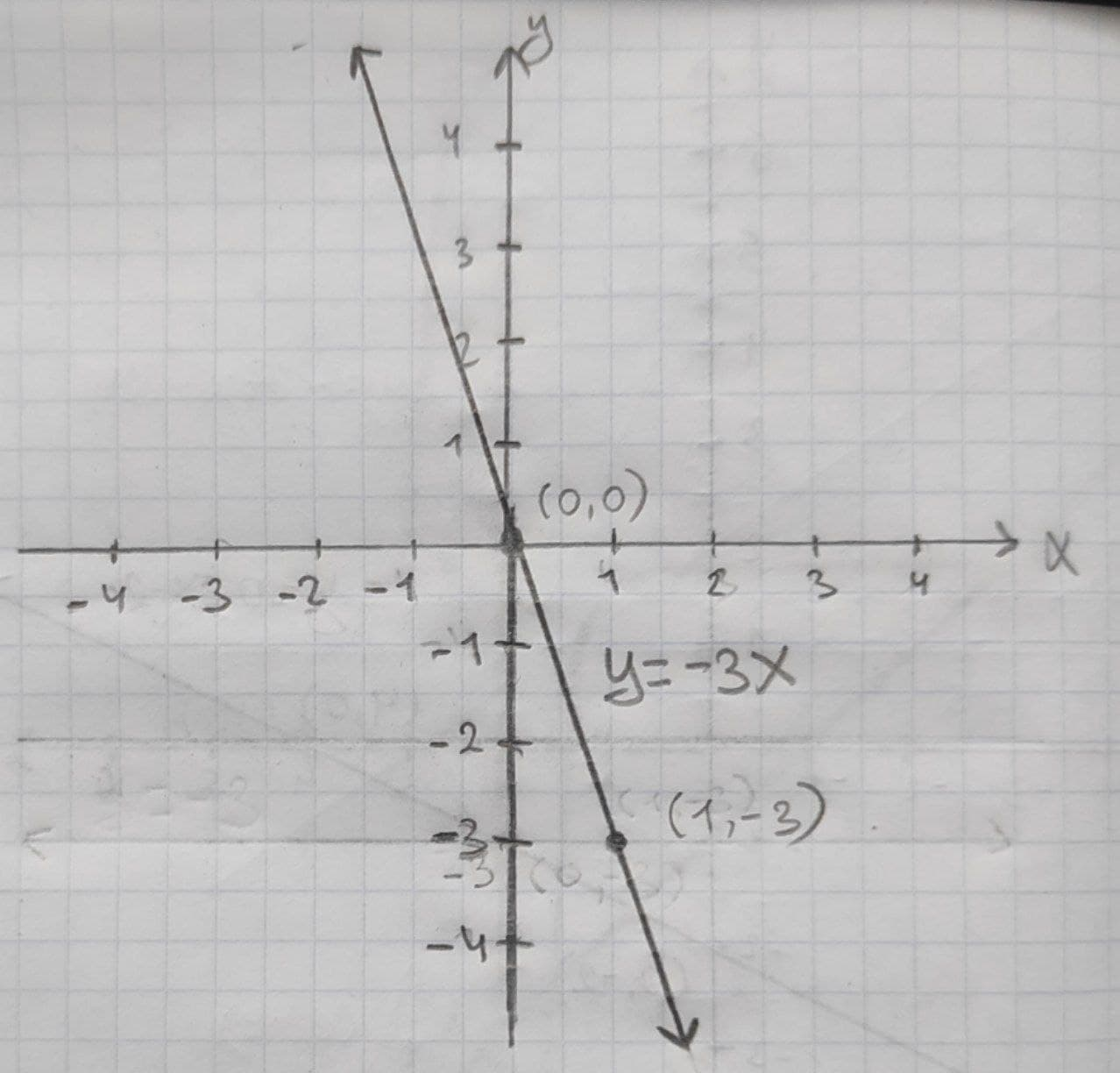cistG

2020-12-06

Given linear equation y = -3x
a. find the y-intercept and slope.
b. determine whether the line slopes upward, slopes downward, or is horizontal, without graphing the equation.
c. use two points to graph the equation.Nathanael Webber

(a) In given example,
y-intercept $={b}_{0}=0$
Slope $={b}_{1}=-3$
(b) When slope is positive then line slope will show upward in a graph. When slope is negative then line slope will show downward in a graph.
Here, slope is -3 which is negative. Hence, line slopes will be downwards in a graph.
(c) Linear regression equation is, y = -3x
Suppose x = 0 then y =0
If x = 1 then y = -3
Therefore, two points are (0,0) and (1,-3)
Following is the graph for above two points:Do you have a similar question?